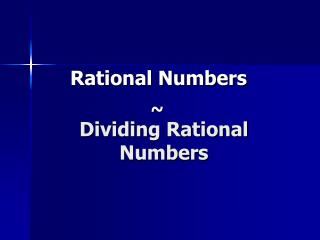Download PresentationDividing Rational Numbers

# Dividing Rational Numbers - PowerPoint PPT PresentationDownload Presentation## Dividing Rational Numbers

- - - - - - - - - - - - - - - - - - - - - - - - - - - E N D - - - - - - - - - - - - - - - - - - - - - - - - - - -
##### Presentation Transcript

1. Rational Numbers ~ Dividing Rational Numbers

2. Dividing Rational Numbers RULES: 1. When multiplying or dividing integers with the same signs, the answer will be Positive.

3. Dividing Rational Numbers RULES: 1. When multiplying or dividing integers with the same signs, the answer will be Positive. 2. When multiplying or dividing integers with different signs, the answer will be Negative.

4. Rules for Dividing Rational Numbers: Dividing two integers with the same signs: ÷ Positive Positive= Positive + + + ÷ = ÷ Negative Negative= Positive + - - + ÷ =

5. Rules for Dividing Rational Numbers: Dividing two integers with different signs: ÷ Positive Negative= Negative - - + ÷ = ÷ NegativePositive= Negative - - + ÷ =

6. -48 ÷ 6= 24 ÷ -6= -63 ÷ -9= Try some. Find the quotient. Remember the rules for dividing rational numbers.

7. Try some. -48 ÷ 6= -8 - - + 24 ÷ -6= -4 - - + -63 ÷ -9= 7 - - +

8. Dividing Rational Numbers

9. Dividing Rational Numbers

10. Dividing Rational Numbers Use the same rules for multiplying as dividing. [Remember that division by zero is undefined.] -32 ÷ 8 = -48 ÷ (-6) = -56 ÷ (-4) = -140 ÷ 5 = -4 8 14 -28

11. Dividing Rational Numbers Example Solve x = -52 ¸ (-4). x = -52 ¸ (-4) The dividend and the divisor have the same sign.

12. Dividing Rational Numbers Example Solve x = -52 ¸ (-4). x = -52 ¸ (-4) x = 13 The dividend and the divisor have the same sign. The quotient is positive.

13. Dividing Rational Numbers • Practice: • 1. -60 ¸ 15

14. Dividing Rational Numbers • Practice: • 1. -60 ¸ 15 -4

15. Dividing Rational Numbers • Practice: • 1. -60 ¸ 15 • 2. 45 ¸ -5

16. Dividing Rational Numbers • Practice: • 1. -60 ¸ 15 • 2. 45 ¸ -5 -9

17. Dividing Rational Numbers • Practice: • 1. -60 ¸ 15 • 2. 45 ¸ -5 • 3. -27 ¸ (-9)

18. Dividing Rational Numbers • Practice: • 1. -60 ¸ 15 • 2. 45 ¸ -5 • 3. -27 ¸ (-9) 3

19. Dividing Rational Numbers • Practice: • 1. -60 ¸ 15 • 2. 45 ¸ -5 • 3. -27 ¸ (-9) • 4. 27 ¸ 9

20. Dividing Rational Numbers • Practice: • 1. -60 ¸ 15 • 2. 45 ¸ -5 • 3. -27 ¸ (-9) • 4. 27 ¸9 3

21. Dividing Rational Numbers • Practice: • 1. -60 ¸ 15 • 2. 45 ¸ -5 • 3. -27 ¸ (-9) • 4. 27 ¸ 9 • 5. 63 ¸ (-3)

22. Dividing Rational Numbers • Practice: • 1. -60 ¸ 15 • 2. 45 ¸ -5 • 3. -27 ¸ (-9) • 4. 27 ¸ 9 • 5. 63 ¸ (-3) -21

23. Student Activity You will now receive a worksheet. Turn the worksheet in when completed.

24. Do Not Disturb Work In Progress